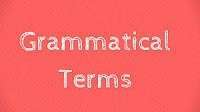# Grammatical Terms

Grammatical Terms are difficult to understand but we are giving such usage grammar that will clarify many of your doubts like grammer or grammar because this is a complete grammar list of items.

Grammatical Terms Starting with A

Grammatical Terms Starting with B & C

Grammatical Terms Starting with D

Grammatical Terms Starting with E & F

Grammatical Terms Starting with G & H

Grammatical Terms Starting with I

Grammatical Terms Starting with K, L & M

Grammatical Terms Starting with N

Grammatical Terms Starting with O & P

Grammatical Terms Starting with Q & R

Grammatical Terms Starting with S

Grammatical Terms Starting with T – Y